## Automating Values from Grouped Data

In the previous page, Getting Measures from Grouped Data, we walked through processes to find values such as the approximate mean and standard deviation from data that is presented in a frequency table of intervals (bins). An example of this situation was given by the table:

Figure 1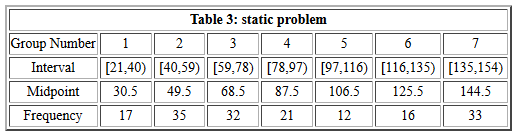The approach that we took involved
1. creating a list of the midpoints of the intervals
2. creating a list of the frequencies of the intervals
3. creating a new valiable that had each midpoint value repeated the frequency number of times.
This process could be used to solve any similar problem. The process never changes. The only change that we have from problem to problem is that we have different low and high points for the intervals, a different number of intervals, and different frequencies for each interval. Because the steps that we take to solve the problem never change we can capture those steps in a function.

When we set out to design such a function we need to determine what values we need to give to the function. Clearly, the frequencies change for each problem and even the number of intervals will change. Also, the low and high ends of each interval change. However, the number of intervals is always equal to the number of frequencies. Thus, if we know the number of frequencies we also know the number of intervals.

In our problems it is always the case that the intervals are of a uniform width, that is, if the first interval is 15 units wide then all of the intervals in that problem are 15 units wide. Therefore, if we know the width of the first interval then we know the width of all the intervals. But all we need to find the width of the first interval is the low and high value for that interval. This means that for the problem shown in Figure 1 above, all of the data for the problem is determined by knowing
1. that the first interval starts at 21
2. that the first interval ends at 40, and
3. that the list of frequencies is 17, 35, 32, 21, 12, 16, 33
From just that information we could construct the rest of Table 3 given in Figure 1.

The function get_from_table() uses that kind of information to produce a few values, and as a side benefit, to create a variable that holds each of the interval midpoints the number of times given by the interval frequency. Figure 2 shows the command to put the function into our environment (line 5) and then to run it with the information given in Figure 1 (line 11). (This does assume that we are running these commands from the subdirectory of the folder that holds all of our functions.)

Figure 2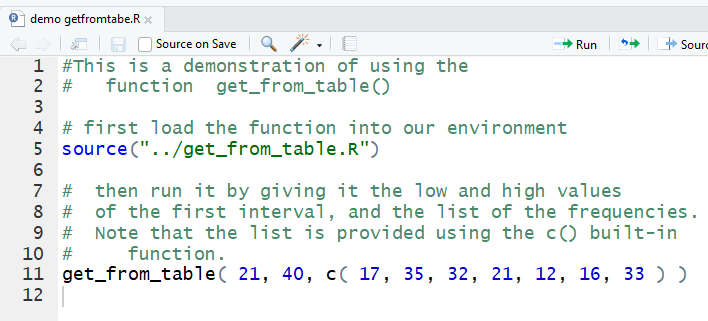The result of running the ommands of Figure 2 are shown in Figure 3.

Figure 3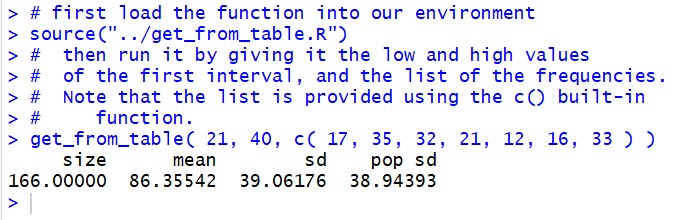We see that `get_from_table()` gives us the number of items given by the frequencies of Table 3 (in this case 166), the approximate mean of the values (86.35542) derived as we had done previously from using the midpoints, the standard deviation if this is a sample (39.06176), and the standard deviation if this is a population (38.94393). In addition, although not shown in the output, `getfromtable()` leaves the created list of midpoint values in a variable called `from_table_x`. We can see that the variable is there by looking at the environment area, shown in Figure 4.

Figure 4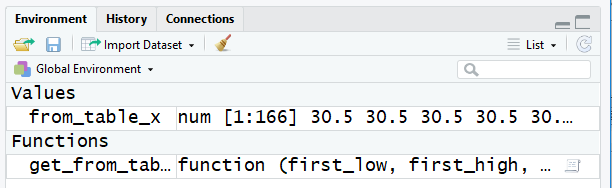Figure 5 shows the result of looking at `from_table_x`.

Figure 5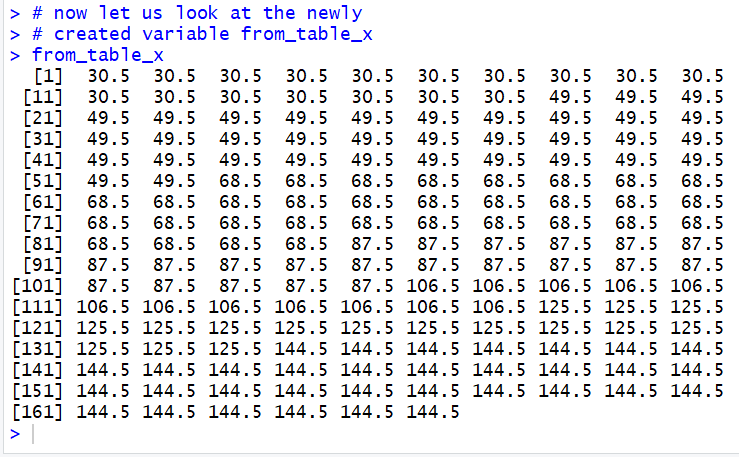Thus we see that 30.5 is the midpoint of the first interval and that the variable `from_table_x` does hold 21 of those values. After those values, `from_table_x` holds 40 of the next midpoint, namely 49.5, and so on.

Because we have access to `from_table_x` we can use it in further computations, such as to find the median of the values. This is shown in Figure 6.

Figure 6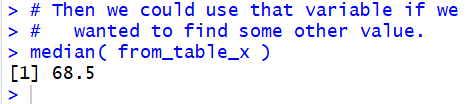It is worth checking all of these results against the values that we worked out, step by step, back in the previous page, Getting Measures from Grouped Data.